# nLab Jacobi diagram

Contents

### Context

#### Graph theory

graph theory

graph

category of simple graphs

### Extra structure

#### Knot theory

knot theory

Examples/classes:

Types

knot invariants

link invariants

Related concepts:

category: knot theory

# Contents

## Idea

A closed Jacobi diagram is a connected undirected graph with oriented trivalent vertices and with an embedded oriented circle, regarded modulo cyclic identifications, if any.

Here is a picture of a typical Jacobi diagram: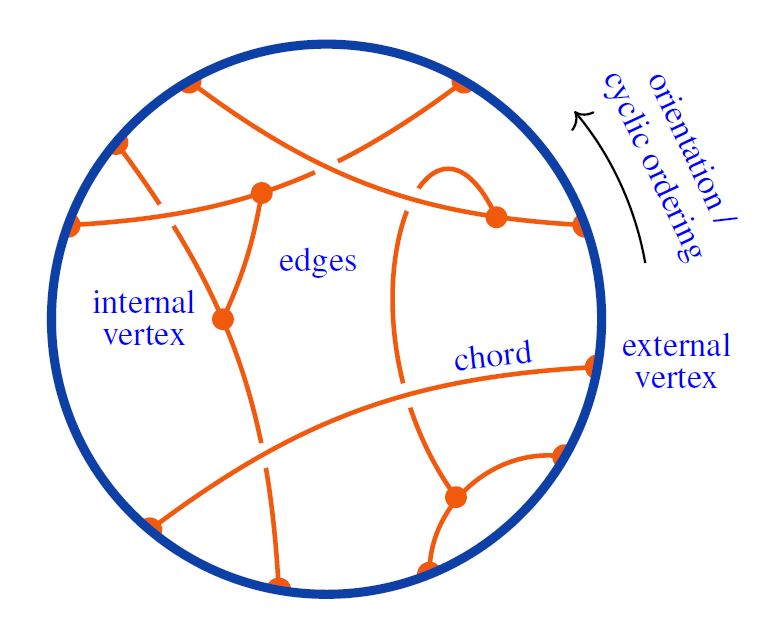Here the internal lines need not form a tree; the following is also a Jacobi diagram: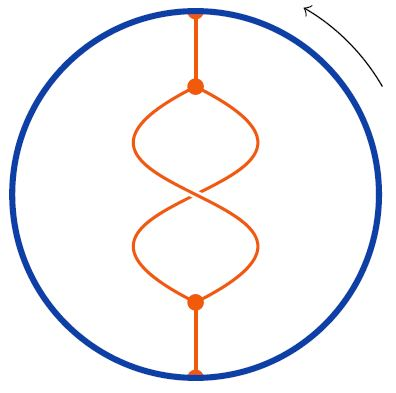graphics from Sati-Schreiber 19c

If all the vertices sit on the circle, Jacobi diagrams specialize to chord diagrams.

Half the number of vertices of a Jacobi diagram is called its order

$order(\Gamma) \;\coloneqq\; \#Vertices(\Gamma)/2$

For example, these are the two closed Jacobi diagrams with 2 vertices (order 1):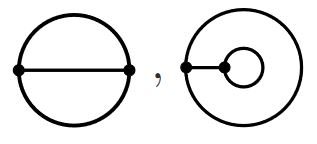and these are are the 10 closed Jacobi diagrams with 4 vertices (order 2):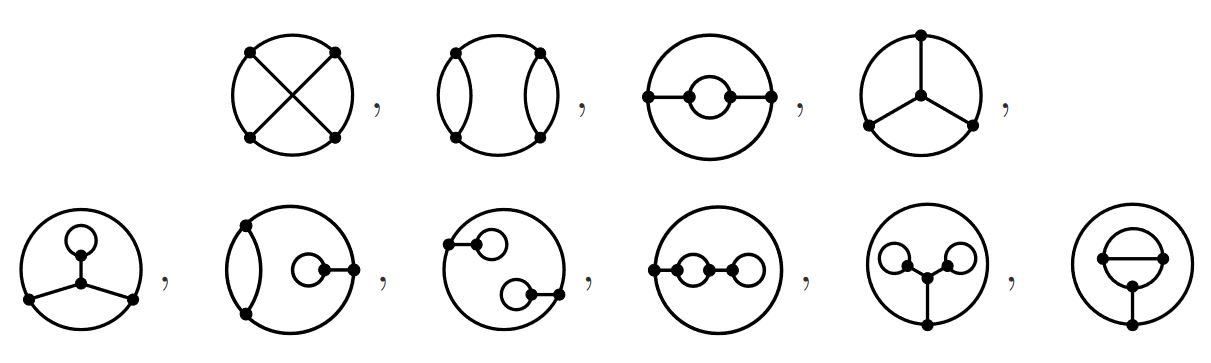graphics grabbed from Chmutov-Duzhin-Mostovoy 11

In applications to Vassiliev invariants in knot theory, Jacobi diagrams play the role of connected Feynman diagrams for Chern-Simons theory in the presence of one Wilson line (the circle is then the knot/Wilson line).

Terminology. Jacobi diagrams have originally been called “chinese character diagrams” (Bar-Natan 95, Def. 1.8) or “Feynman diagrams for Chern-Simons theory” (Kricker-Spence-Aitchison 97) or “circle diagrams” (Kneissler 97) or “round diagrams” (Willerton 99). The term “Jacobi diagram” (Chmutov-Duzhin-Mostovoy 11, Chapter 5, Jackson-Moffat 19, Section 13) alludes to the Jacobi identity – here also called the STU relation – via the fact that these diagrams are naturally labelled by a Lie algebra equipped with a Lie algebra representation (which is really just the interaction-aspect of their interpretation as Chern-Simons theory Feynman diagrams…).

## Definition

(…)

### STU-relations and weight systems

For $R \in$ CRing a commutative ring, let $R\langle \mathcal{D}^t \rangle$ denote the $R$-linear span of the set $\mathcal{D}^t$ of Jacobi diagrams.

Then one traditionally writes

(1)$\mathcal{A}^t \;\coloneqq\; R\langle \mathcal{D}^c \rangle/STU$

for the quotient spaces of the linear span of Jacobi diagrams by the STU relations: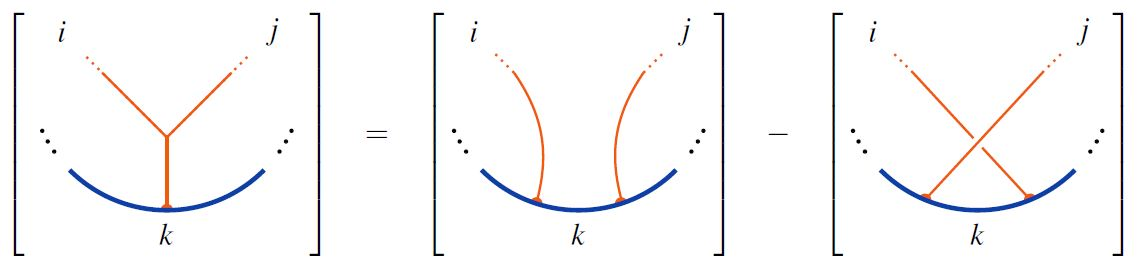Hence: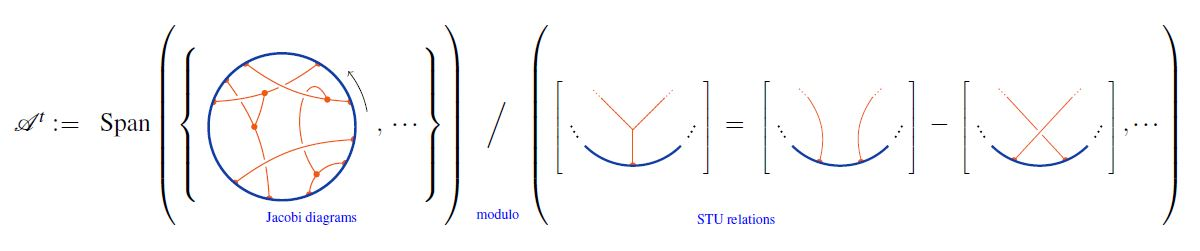$w \;\colon\; \mathcal{A}^t \longrightarrow R \,,$

– hence a plain $R$-valued function on the set $\mathcal{D}^t$ of Jacobi diagrams which is invariant under the STU relations

is called a framed weight system. See there for more.

graphics from Sati-Schreiber 19c

## Properties

### Relation to chord diagrams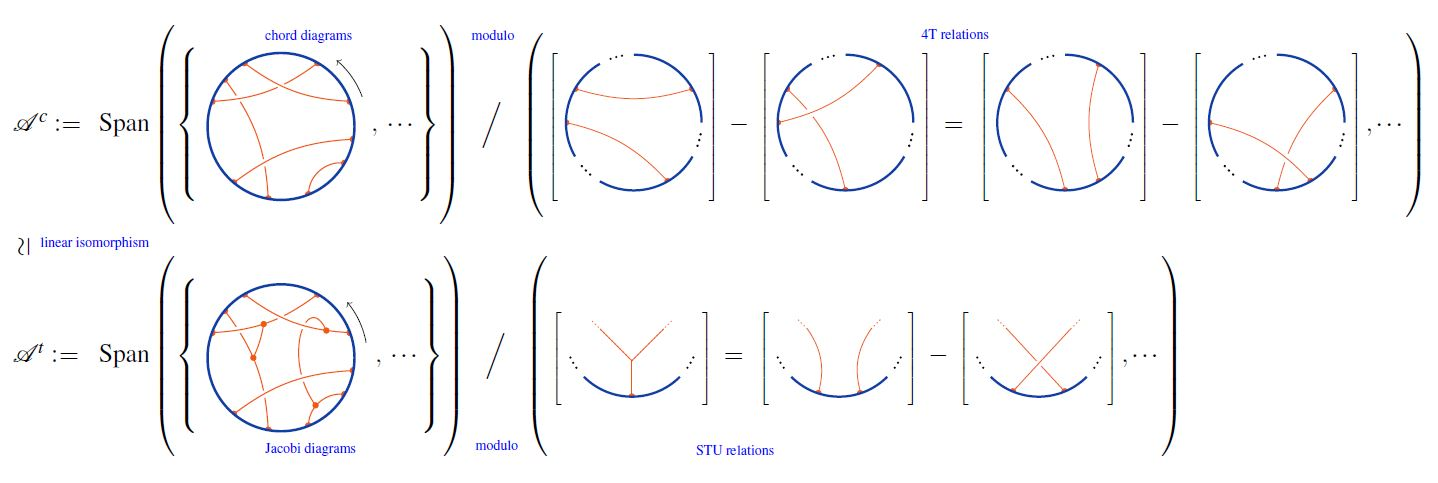graphics from Sati-Schreiber 19c

### Relation to Adams operations

On the vector space $\mathcal{A}$ of Jacobi diagrams modulo STU-relations (equivalently chord diagrams modulo 4T relations) there is a system of linear maps (for $q \in \mathbb{Z}$, $q \neq 0$)

$\psi^q \;\colon\; \mathcal{A} \longrightarrow \mathcal{A}$

which respect the coalgebra structure and satisfy

$\psi^{q_2} \circ \psi^q_1 \;=\; \psi^{q_1 \cdot q_2}$

and as such are (dually) analogous to the Adams operations on topological K-theory.

In fact, when evaluated in Lie algebra weight systems $w_{\mathbf{N}}$ and under the identification (see here) of the representation ring of a compact Lie group $G$ with the $G$-equivariant K-theory of the point, these Adams operations on Jacobi diagrams correspond to the Adams operations on equivariant K-theory:

$w_{\mathbf{N}}(\psi^q D) \;=\; w_{\psi^q \mathbf{N}}(D) \,.$

For more see at Adams operation on Jacobi diagrams.

### General

Original articles

• Dror Bar-Natan, On the Vassiliev knot invariants, Topology Volume 34, Issue 2, April 1995, Pages 423-472 (doi:10.1016/0040-9383(95)93237-2, pdf)

• A. Kricker, B. Spence and I. Aitchison, Cabling the Vassiliev invariants, J. Knot Theory Ramifications 6 (1997) 327–358

• Jan Kneissler, The number of primitive Vassiliev invariants up to degree 12 (arXiv:q-alg/9706022)

• Simon Willerton, The Kontsevich integral and algebraic structures on the space of diagrams in: Knots in Hellas ’98. Series on Knots and Everything, vol. 24, World Scientific, 2000, 530–546 (arXiv:math/9909151)

Lecture notes

Textbook accounts

### Chord diagrams and weight systems in Physics

The following is a list of references that involve (weight systems on) chord diagrams/Jacobi diagrams in physics:

For a unifying perspective and further pointers see:

#### In Chern-Simons theory

Since weight systems are the associated graded of Vassiliev invariants, and since Vassiliev invariants are knot invariants arising as certain correlators/Feynman amplitudes of Chern-Simons theory in the presence of Wilson lines, there is a close relation between weight systems and quantum Chern-Simons theory.

Historically this is the original application of chord diagrams/Jacobi diagrams and their weight systems, see also at graph complex and Kontsevich integral.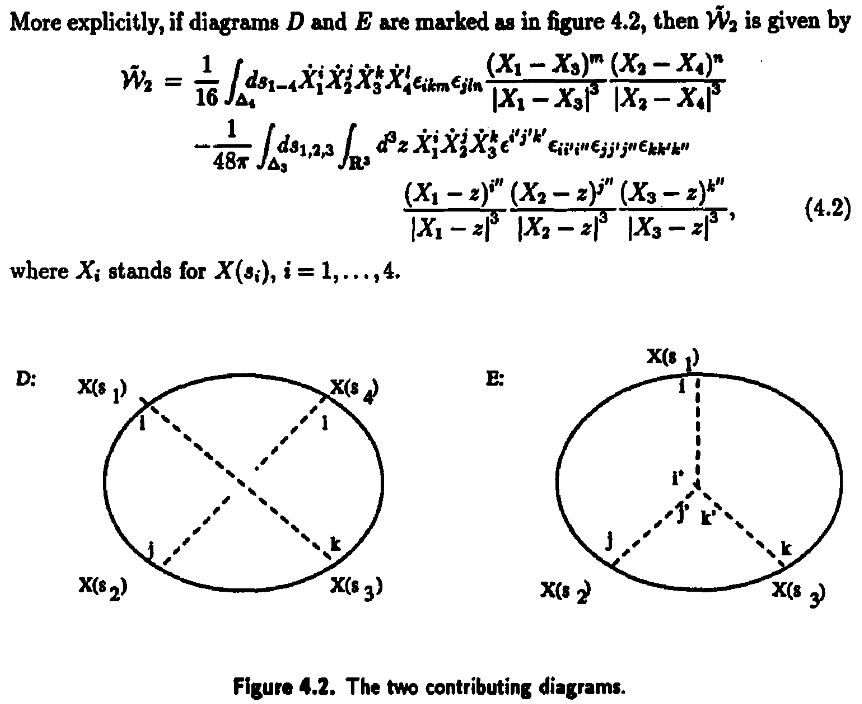Reviewed in:

Applied to Gopakumar-Vafa duality:

• Dave Auckly, Sergiy Koshkin, Introduction to the Gopakumar-Vafa Large $N$ Duality, Geom. Topol. Monogr. 8 (2006) 195-456 (arXiv:0701568)

See also

#### For single trace operators in AdS/CFT duality

Interpretation of Lie algebra weight systems on chord diagrams as certain single trace operators, in particular in application to black hole thermodynamics

#### In $AdS_2/CFT_1$, JT-gravity/SYK-model

Discussion of (Lie algebra-)weight systems on chord diagrams as SYK model single trace operators:

• Antonio M. García-García, Yiyang Jia, Jacobus J. M. Verbaarschot, Exact moments of the Sachdev-Ye-Kitaev model up to order $1/N^2$, JHEP 04 (2018) 146 (arXiv:1801.02696)

• Yiyang Jia, Jacobus J. M. Verbaarschot, Section 4 of: Large $N$ expansion of the moments and free energy of Sachdev-Ye-Kitaev model, and the enumeration of intersection graphs, JHEP 11 (2018) 031 (arXiv:1806.03271)

• Micha Berkooz, Prithvi Narayan, Joan Simón, Chord diagrams, exact correlators in spin glasses and black hole bulk reconstruction, JHEP 08 (2018) 192 (arxiv:1806.04380)

following:

• László Erdős, Dominik Schröder, Phase Transition in the Density of States of Quantum Spin Glasses, D. Math Phys Anal Geom (2014) 17: 9164 (arXiv:1407.1552)

which in turn follows

• Philippe Flajolet, Marc Noy, Analytic Combinatorics of Chord Diagrams, pages 191–201 in Daniel Krob, Alexander A. Mikhalev,and Alexander V. Mikhalev, (eds.), Formal Power Series and Algebraic Combinatorics, Springer 2000 (doi:10.1007/978-3-662-04166-6_17)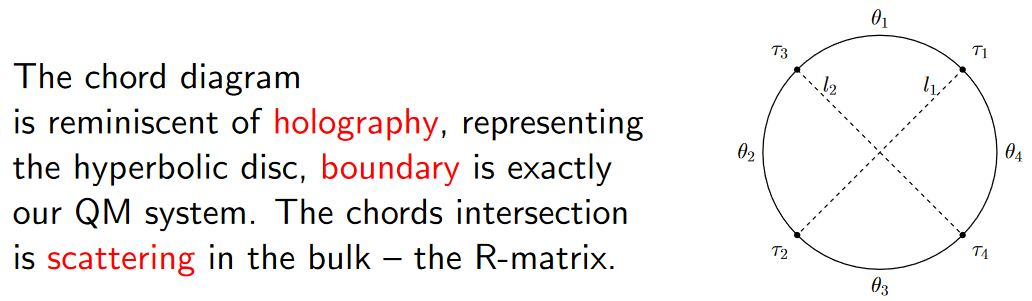With emphasis on the holographic content:

• Micha Berkooz, Mikhail Isachenkov, Vladimir Narovlansky, Genis Torrents, Section 5 of: Towards a full solution of the large $N$ double-scaled SYK model, JHEP 03 (2019) 079 (arxiv:1811.02584)

• Vladimir Narovlansky, Slide 23 (of 28) of: Towards a Solution of Large $N$ Double-Scaled SYK, 2019 (pdf)

and specifically in relation, under AdS2/CFT1, to Jackiw-Teitelboim gravity:

#### In D$p$/D$(p+2)$-brane intersections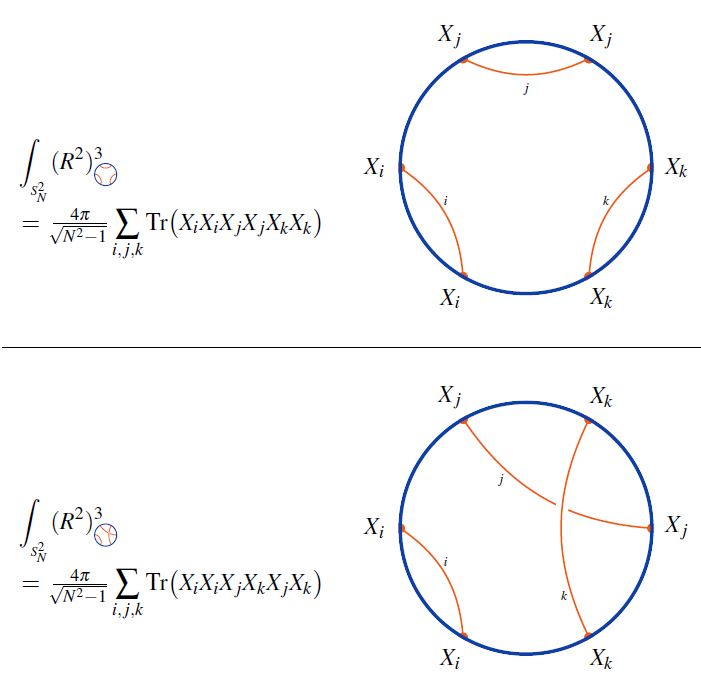graphics from Sati-Schreiber 19c

#### As codes for holographic entanglement entropyFrom Yan 20

Chord diagrams encoding Majorana dimer codes and other quantum error correcting codes via tensor networks exhibiting holographic entanglement entropy:From JGPE 19

#### For Dyson-Schwinger equations

Discussion of round chord diagrams organizing Dyson-Schwinger equations:

• Nicolas Marie, Karen Yeats, A chord diagram expansion coming from some Dyson-Schwinger equations, Communications in Number Theory and Physics, 7(2):251291, 2013 (arXiv:1210.5457)

• Markus Hihn, Karen Yeats, Generalized chord diagram expansions of Dyson-Schwinger equations, Ann. Inst. Henri Poincar Comb. Phys. Interact. 6 no 4:573-605 (arXiv:1602.02550)

Review in:

• Ali Assem Mahmoud, Section 3 of: On the Enumerative Structures in Quantum Field Theory (arXiv:2008.11661)